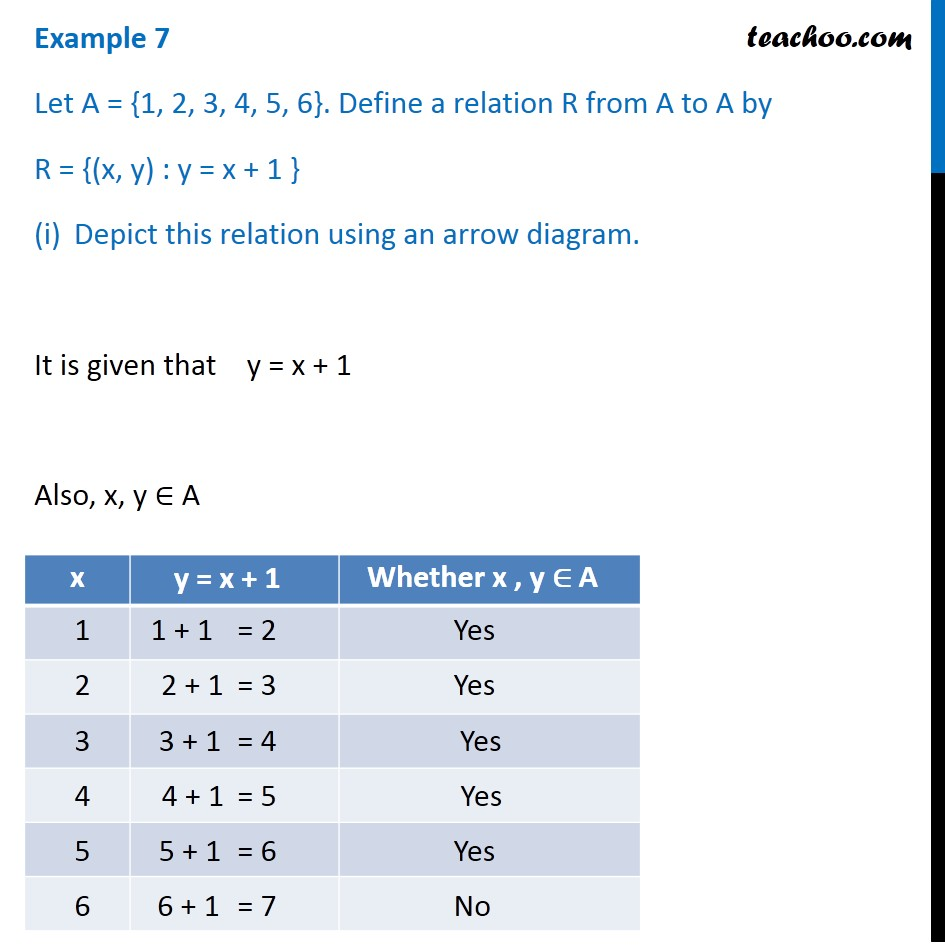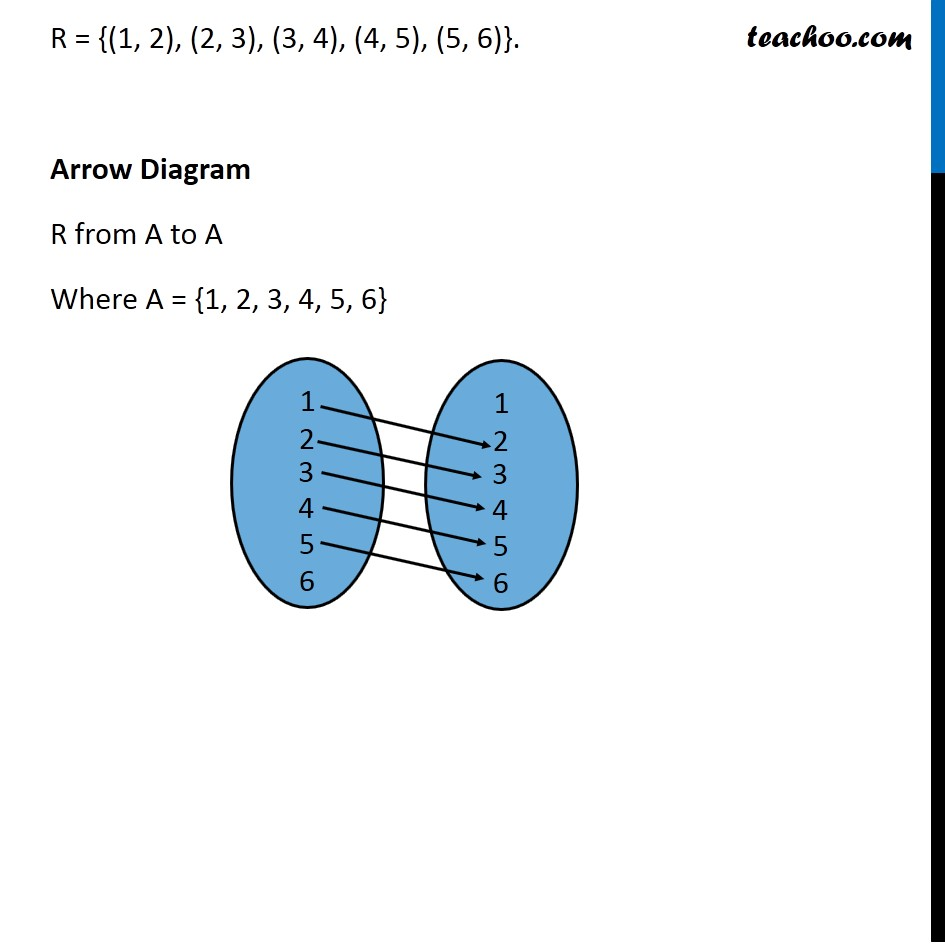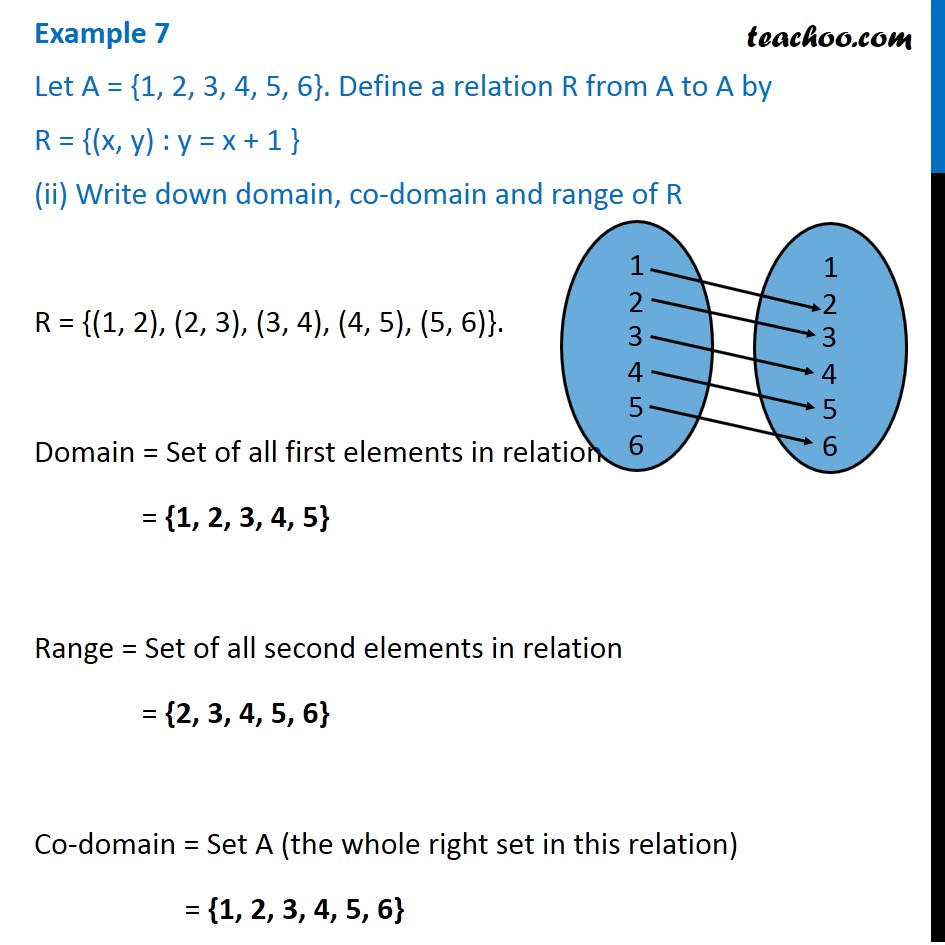Finding Relation - Set-builder form given

Chapter 2 Class 11 Relations and Functions (Term 1)
Concept wise### Transcript

Example 7 Let A = {1, 2, 3, 4, 5, 6}. Define a relation R from A to A by R = {(x, y) : y = x + 1 } Depict this relation using an arrow diagram. It is given that y = x + 1 Also, x, y ∈ A R = {(1, 2), (2, 3), (3, 4), (4, 5), (5, 6)}. Arrow Diagram R from A to A Where A = {1, 2, 3, 4, 5, 6} Example 7 Let A = {1, 2, 3, 4, 5, 6}. Define a relation R from A to A by R = {(x, y) : y = x + 1 } (ii) Write down domain, co-domain and range of R R = {(1, 2), (2, 3), (3, 4), (4, 5), (5, 6)}. Domain = Set of all first elements in relation = {1, 2, 3, 4, 5} Range = Set of all second elements in relation = {2, 3, 4, 5, 6} Co-domain = Set A (the whole right set in this relation) = {1, 2, 3, 4, 5, 6}